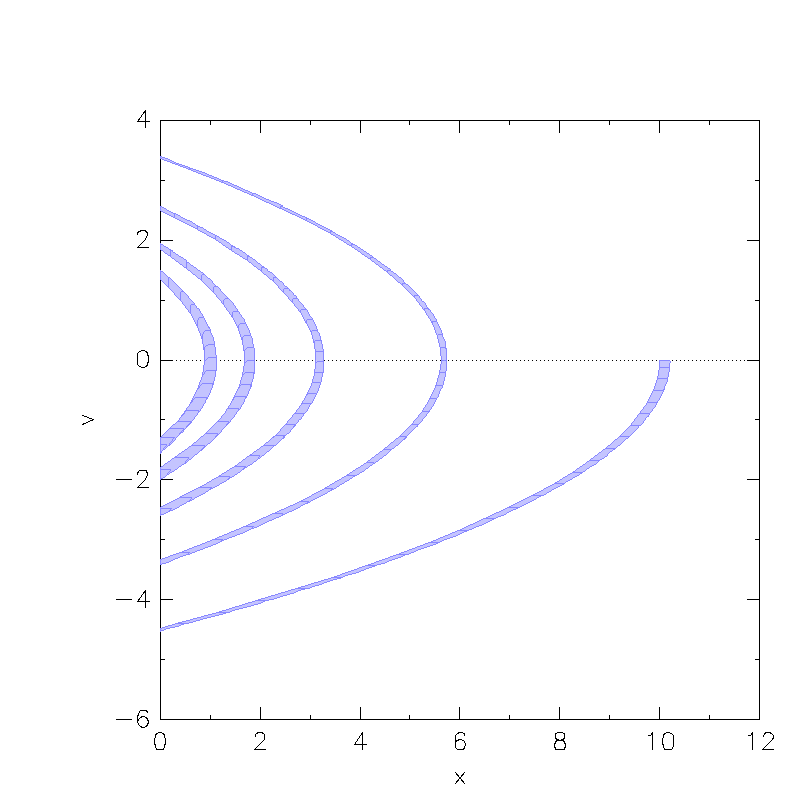## Classification

# of variables # of modes # of jumps
2 1 1
Type Continuous dynamics Guards & Invariants Resets
hybrid linear polynomial half-space linear

 Flow* flow*_files SpaceEx spaceEx_files

## Model description

The classical bouncing ball example consists of a ball dropped from a predefined height. It hits the ground after a certain time, loses energy and then bounces back into the air and starts to fall again. This physical phenomena can be represented as the following hybrid automaton: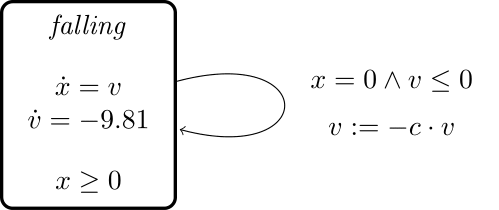In the discrete state, the motion of the ball, assumed to have a mass of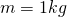, is governed by the following differential equation: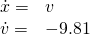where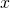is the ball’s height from the ground,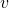is the ball’s vertical velocity, and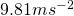is the earth’s gravitational force. The invariant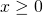enforces that the ball always bounces when it reaches the ground. The guard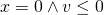of the single discrete transition, which models the bouncing, ensures that bouncing happens after falling when reaching the ground. The corresponding reset condition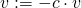accounts for the loss of energy due to the ball’s deformation, where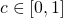is a constant.

## Reachability settings

We consider the inital set

•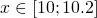•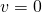.

We use a time horizon of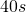and set the constant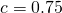. The set of bad states is the set of all states where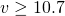.

## Results

These are the results from the bouncing ball benchmark created with SpaceEx: